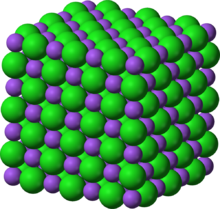# Lattice energy

﻿
Lattice energySodium chloride crystal lattice

The lattice energy of an ionic solid is a measure of the strength of bonds in that ionic compound. It is usually defined as the enthalpy of formation of the ionic compound from gaseous ions and as such is invariably exothermic. Lattice energy may also be defined as the energy required to completely separate one mole of a solid ionic compound into gaseous ionic constituents. The concept of lattice energy was initially developed for rocksalt-structured and sphalerite-structured compounds like NaCl and ZnS, where the ions occupy high-symmetry crystal lattice sites. In the case of NaCl, the lattice energy is the energy released by the reaction

Na+ (g) + Cl (g) → NaCl (s)

which would amount to -787 kJ/mol.

Some older textbooks define lattice energy as the energy required to convert the ionic compound into gaseous ions which is an endothermic process, and following this definition the lattice energy of NaCl would be +787 kJ/mol.

The precise value of the lattice energy may not be determined experimentally, because of the impossibility of preparing an adequate amount of gaseous anions and cations and measuring the energy released during their condensation to form the solid. However, the value of the lattice energy may either be derived theoretically from electrostatics or from a thermodynamic cycling reaction, the so-called Born-Haber cycle.

## Theoretical treatments

### Born-Landé equation

In 1918 Born and Landé proposed that the lattice energy could be derived from the electric potential of the ionic lattice and a repulsive potential energy term.$E = -\frac{N_AMz^+z^- e^2 }{4 \pi \varepsilon_0 r_0}\left(1-\frac{1}{n}\right),$

where

M is the Madelung constant, relating to the geometry of the crystal;
z+ is the charge number of cation;
z is the charge number of anion;
e is the elementary charge, equal to 1.6022×10−19 C;
ε0 is the permittivity of free space, equal to 8.854×10−12 C2 J−1 m−1;
r0 is the distance to closest ion; and
n is the Born exponent, a number between 5 and 12, determined experimentally by measuring the compressibility of the solid, or derived theoretically.

The Born-Landé equation gives a reasonable fit to the lattice energy.

Compound Calculated Lattice Energy Experimental Lattice Energy
NaCl −756 kJ/mol −787 kJ/mol
LiF −1007 kJ/mol −1046 kJ/mol
CaCl2 −2170 kJ/mol −2255 kJ/mol

From the Born-Landé equation it can be seen that the lattice energy of a compound is dependent on a number of factors

• as the charges on the ions increase the lattice energy increases (becomes more negative),
• when ions are closer together the lattice energy increases (becomes more negative)

Barium oxide (BaO), for instance, which has the NaCl structure and therefore the same Madelung constant, has a bond radius of 275 picometers and a lattice energy of -3054 kJ/mol, while sodium chloride (NaCl) has a bond radius of 283 picometers and a lattice energy of -786 kJ/mol.

### Kapustinskii equation

The Kapustinskii equation can be used as a simpler way of deriving lattice energies where high precision is not required.

### Effect of polarisation

For ionic compounds with ions occupying lattice sites with crystallographic point groups C1, C1h, Cn or Cnv (n = 2, 3, 4 or 6) the concept of the lattice energy and the Born-Haber cycle has to be extended. In these cases the polarization energy Epol associated with ions on polar lattice sites has to be included in the Born-Haber cycle and the solid formation reaction has to start from the already polarized species. As an example, one may consider the case of iron-pyrite FeS2, where sulfur ions occupy lattice site of point symmetry group C3. The lattice energy defining reaction then reads

Fe2+ (g) + 2 pol S (g) → FeS2 (s)

where pol S stands for the polarized, gaseous sulfur ion. It has been shown that the neglection of the effect led to 15 % difference between theoretical and experimental thermodynamic cycle energy of FeS2 that reduced to only 2%, when the sulfur polarization effects were included.

Wikimedia Foundation. 2010.

### Look at other dictionaries:

• lattice energy — noun (chem) Energy required to separate the ions of a crystal from each other to an infinite distance • • • Main Entry: ↑lattice …   Useful english dictionary

• lattice energy — ▪ crystals       the energy needed to completely separate an ionic solid, such as common table salt, into gaseous ions (ion) (also the energy released in the reverse process). Lattice energy is usually measured in kilojoules per mole (1 mole =… …   Universalium

• lattice energy — gardelės energija statusas T sritis Standartizacija ir metrologija apibrėžtis Energijos kiekis, išsiskiriantis susidarant iš dujinėje fazėje esančių jonų vienai kristalinės medžiagos molekulei (moliui). atitikmenys: angl. lattice energy vok.… …   Penkiakalbis aiškinamasis metrologijos terminų žodynas

• lattice energy — gardelės energija statusas T sritis Standartizacija ir metrologija apibrėžtis Kristalo gardelę sudarančių dalelių ryšio energija. atitikmenys: angl. lattice energy vok. Gitterenergie, f rus. энергия решетки, f pranc. énergie réticulaire, f …   Penkiakalbis aiškinamasis metrologijos terminų žodynas

• lattice energy — gardelės energija statusas T sritis chemija apibrėžtis Energijos kiekis, išsiskiriantis susidarant iš dujinėje fazėje esančių jonų vienai kristalinės medžiagos molekulei (moliui). atitikmenys: angl. lattice energy rus. энергия решетки …   Chemijos terminų aiškinamasis žodynas

• lattice energy — gardelės energija statusas T sritis fizika atitikmenys: angl. lattice energy vok. Gitterenergie, f rus. энергия решётки, f pranc. énergie du réseau, f; énergie réticulaire, f …   Fizikos terminų žodynas

• lattice energy — noun The energy required to separate the ions of an ionic solid (especially a crystal) to infinity …   Wiktionary

• lattice energy — Смотри энергия кристаллической решетки …   Энциклопедический словарь по металлургии